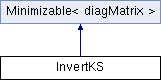JDFTx  1.7.0InvertKS Class Reference

Invert Kohn-Sham equations to get effective potential for a given electron density. More...

#include <RadialSchrodinger.h>

Inheritance diagram for InvertKS:## Public Member Functions

Initialize for a given target electron density nTarget.

Get the orbital KE density.

void step (const diagMatrix &dV, double alpha)
Move the state in parameter space along direction dir with scale alpha.

double compute (diagMatrix *E_V, diagMatrix *KE_V)Public Member Functions inherited from Minimizable< diagMatrix >
virtual bool report (int iter)

virtual void constrain (diagMatrix &)
Constrain search directions to the space of free directions for minimize.

virtual double sync (double x) const
Override to synchronize scalars over MPI processes (if the same minimization is happening in sync over many processes)

virtual double safeStepSize (const diagMatrix &dir) const
Override to return maximum safe step size along a given direction. Steps can be arbitrarily large by default.

double minimize (const MinimizeParams &params)
Minimize this objective function with algorithm controlled by params and return the minimized value.

void fdTest (const MinimizeParams &params)

## Detailed Description

Invert Kohn-Sham equations to get effective potential for a given electron density.

The documentation for this class was generated from the following file: